# High School Math : Understanding Absolute Value

## Example Questions

### Example Question #1 : Understanding Absolute Value

Expression 1: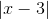Expression 2: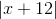Find the set of values forwhere Expression 1 is greater than Expression 2.

Possible Answers:

All values where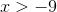All values where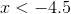All values where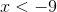All real numbers

All values where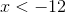Correct answer:

All values whereExplanation:

In finding the values forwhere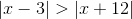, break the comparison of these two absolute value expressions into the four possible ways this could potentially be satisfied.

The first possibility is described by the inequality:If you think of a number line, it is evident that there is no solution to this inequality since there will never be a case where subtracting fromwill lead to a greater number than adding to.

The second possibility, wherein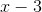is negative and converted to its opposite to being an absolute value expression but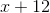is positive and requires no conversion, can be represented by the inequality (where the sign is inverted due to multiplication by a negative):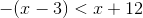We can simplify this inequality to find thatsatisfies the conditions where.

The third possibility can be represented by the following inequality (where the sign is inverted due to multiplication by a negative):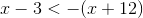This is again simplified toand is redundant with the above inequality.

The final possibility is represented by the inequality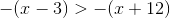This inequality simplifies to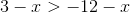. Rewriting this as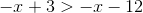makes it evident that this inequality is true of all real numbers. This does not provide any additional conditions on how to satisfy the original inequality.

The only possible condition that satisfies the inequality is that which arises in two of the tested cases, when.

### Example Question #2 : Understanding Absolute Value

What is the absolute value of -3?

Possible Answers:

10

-3

1

3

9

Correct answer:

3

Explanation:

The absolute value is the distance from a given number to 0. In our example, we are given -3. This number is 3 units away from 0, and thus the absolute value of -3 is 3.

If a number is negative, its absolute value will be the positive number with the same magnitude. If a number is positive, it will be its own absolute value.

### All High School Math Resources